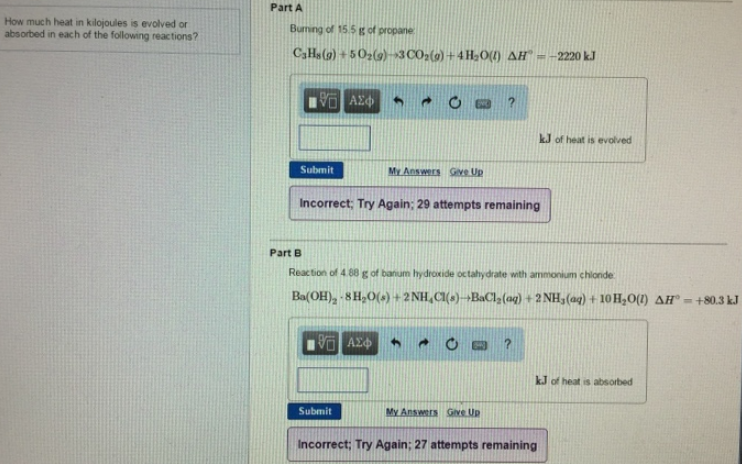# Problem: How much heat in kilojoules is evolved or absorbed in each of the following reactions?Burning of 15.5 g of propane:C3H8(g) + 5 O2(g) → 3 CO2(g) + CO2(g) + 4H2O(l) ΔH°= -2220 kJReaction of 4.88 g of hydroxide with ammonium chloride.Ba(OH)2 •8H2O(s) + 2NH4Cl(s)→ BaCl2(aq) + 2 NH3(aq) + 10H2O(l) ΔH° = +80.3 kJ

###### FREE Expert Solution
100% (445 ratings)###### Problem Details

How much heat in kilojoules is evolved or absorbed in each of the following reactions?

Burning of 15.5 g of propane:

C3H8(g) + 5 O2(g) → 3 CO2(g) + CO2(g) + 4H2O(l) ΔH°= -2220 kJ

Reaction of 4.88 g of hydroxide with ammonium chloride.

Ba(OH)2 •8H2O(s) + 2NH4Cl(s)→ BaCl2(aq) + 2 NH3(aq) + 10H2O(l) ΔH° = +80.3 kJ